MOTION AND MEASUREMENT OF DISTANCES
Motion and Measurement of Distances

1.    In ancient time man used to move only on foot and carry goods either on his back or on the back of some animals.

2.
A great change in the modes of transport was made :
(i)      by the invention of wheel.
(ii)    by the invention of steam engine.

3.
Measurement : It is the technique developed for correct judgement of dimensions of various objects.

4.
Non-standard measures : The lengths of steps, arms, hands, or fingers of different people are different, therefore the distance measured with their help is not always reliable. These methods are therefore called non-standard measures.

5.    Standard measures : Measures that are the same all over the world are known as standard measures.

6.
SI units : In October 1960, the XIIth general conference on weight and measures adopted in International system of units. “The System International Units” is the set of units to maintain uniformity all over the world.

7.
Metre : It is the standard unit of length. The symbol of metre is m.

Each metre (m) is divided into 100 equal divisions, called centimeter (cm). Each
Centimeter has ten equal divisions, called millimeter (mm). Thus
1 m = 100 cm
1 cm = 10 mm
For measuring large distances, metre is not a convenient unit. We define a larger
unit of length. It is called kilometer (km).
1 km = 1000 m.

8.
Simple multiples of units : Units used for the measurement of larger distances are the multiples of SI unit. For example : deca, hector, kilo.
1 decametre = 10 m
1 hectometre = 100 m
1 kilometre = 1000 m

9.
Sub-multiples of units : Units used for measuring smaller distances are the submultiples of SI units.
For example, milli, centi, deci.
1 m = 10 decimetre
1 m = 100 centimetre
1 m = 1000 millimetre.

10.
Making measurement of a length : In making measurement of length of an object we should follow the following procedure :
(1)  Place the scale in contact with the object along its length.

(2)  Measurement with a scale with broken ends
(i)    Avoid taking measurements from zero mark.
(ii)  Use any other full mark of the scale, say 1.0 cm [Fig. 10.4 (a)].
(iii)Subtract the reading of this mark from the reading at the other end. For example, in Fig. 10.4 (b), the reading at starting mark is 1.0 cm and at the other end it is 6.5 cm. Therefore, the length of the object is (6.5 – 1.0) cm = 15.5 cm.

(3)  Correct position of the eye is also important for making measurement. Your eye must be exactly above the point where the measurement is to be taken. Position ‘A’ is the correct position of the eye. Note that from position ‘A’, the reading is 1.0 cm. From positions ‘B’ and ‘C’, the readings may be different.

11.Least count : A scale is marked in centimeters and millimeters . With the scales of this kind we can measure correctly upto one millimeter, that is one-tenth of a centimeter. This is called the least count of a (15 cm) scale.

12.Measuring the length of a curved line : We can not measure the length of a curved line directly by using a metre scale. We can use a thread or divider to measure the length of a curved line.

13.
Motion : It is a state of objects in which they are changing their place with time.

14.
Rest : All the stationary objects which are not in motion, that is, do not change their place with time are said to be at rest.

15.
Rectilinear motion : When the objects change their position with time along a straight line, this type of motion is called rectilinear motion.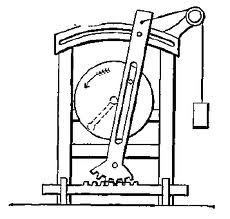16.Circular motion :

(i)    When a body moves in a circular path, its motion is known as circular motion.
(ii)  Example : i. motion of stone tied in a thread and whirled.
ii. motion of a blade of an electric fan.
iii. motion of second’s hand in a clock.
(iii)In circular motion, the object remains at the same distance from a fixed point.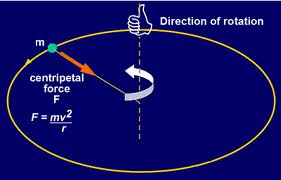17.
Rotational motion : Motion in which a whole body moves about an axis is called a rotational motion. Example : motion of a top.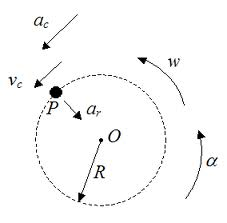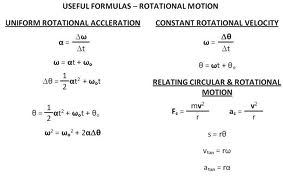18.
Periodic motion : Motion in which an object repeats its motion after a fixed interval of time is called periodic motion.
Example : (i) Oscillations of a pendulum.
(ii) Motion of a swing.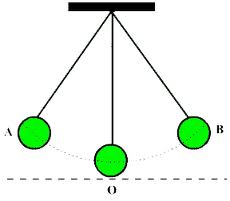19.Combination of two or more types of motions : In some situations, the motion of an object may be a combination of two or more of the above mentioned types of motion. For example,
(i)    Motion of a ball on the ground. Here, the ball is rotating about an axis but the axis itself is moving along a straight line. Thus, the ball executes a rectilinear motion as well as rotational motion.
(ii)  Motion of earth – earth executes rotations on its axis and also revolves around the sun.

20.
Distance : The amount of space between two places is called distance.

21.
Unit of measurements

(i)      It involves the comparison of an unknown quantity with some known quantity of the same kind.
(ii)    This known fixed quantity is called unit.
(iii)   The result of measurement is expressed in two parts. One part is a number. The other part is the unit of measurement.

Q.1. Give two examples each, of modes of transport used on land, water and air.
Ans. (i)   Land – Train, Bus, Motor Bike, Car
(ii)  Water – Ship, Boat
(iii) Air – Aeroplane, Helicopter.

Q.2. Fill in the blanks :

(i)        One metre is ………. cm.
(ii)      Five kilometer is ………. m.
(iii)     Motion of a child on a swing is ……… .
(iv)     Motion of the needle of a sewing machine is ………. .
(v)      Motion of wheel of a bicycle is ……….. .

Ans.
(i)    100
(ii)   5000
(iii)  Periodic (oscillatory motion)
(iv)  Periodic motion
(v)  Circular motion.

Q.3. Why can a pace or a footstep not be used as a standard unit of length?

Ans.
Pace or footstep as a unit of length is not suitable. As their measure is not fixed and varies from person to person. Since a standard unit must be similar in all places of world and in all weathers. It should not depend upon body parts and natural phenomenon.

Q.4. Arrange the following lengths in their increasing magnitude:

1 metre, 1 centimetre, 1 kilometre, 1 millimetre.

Ans.
1 millimetre, 1 centimetre, 1 metre, 1 kilometre.

Q.5. The height of a person is 1.65 m. Express it into cm and mm.

Ans.
Given that, height of person = 1.65 m
We have to find out (a) height in cm
(b) height in mm.
(a) We know that,
1 m = 100 cm
Hence,  1.65 m = 1.65 × 100 cm
= 165 cm. Ans.
(b) Again we know that,
1 m = 1000 mm
Hence,  1.65 m = 1.65 × 1000 mm
= 1650 mm. Ans.
Or
We known that,
1 cm = 10 mm
Hence,    165 cm = 165 × 10 mm
= 1650 mm. Ans.

Q.6. The distance between Radha’s home and her school is 3250 m. Express this distance into km.

Ans.
Given that,
Distance between Radha’s home and her school = 3250 m
We have to find out distance in km.
We know that,
1000 m = 1 km
Hence,           3250 m =km
= 3.250 km. Ans.
Q.7. While measuring the length of a knitting needle, the reading of the scale at one end is 3.0 cm and at the other end is 33.1 cm. What is the length of the needle?

Ans.
Given that,
Reading at starting end = 3.0 cm
Reading at other end = 33.1 cm
We know that,
Length of the object = (Reading at other end – Reading at starting end)
Hence, Length of the needle = 33.1 – 3.0 cm
= 30.1 cm Ans.

Q.8. Write the similarities and differences between the motion of a bicycle and a ceiling fan that has been switched on.

Ans. (i) Similarity :
Both the wheel of a bicycle and a ceiling fan execute a circular motion on a fixed axis.
(ii) Dissimilarity : A bicycle also executes a rectilinear motion while a fan does not execute such motion.

Q.9. Why could you not use an elastic measuring tape to measure distance? What would be some of the problems you would meet in telling someone about a distance you measured with an elastic tape?

Ans.
Elastic substances have no fixed length. Their length is altered when they are stretched by different force. Hence, an elastic tape will not give a correct measurement. This is why, we can not use an elastic measuring tape. As it is very much difficult to measure the amount by which it alters the measure we cannot tell correctly a distance by measuring with an elastic tape.

Q.10. Give two examples of periodic motion.

Ans.
Two examples of periodic motion are :
(i)        Motion of the earth around the sun.
(ii)      Oscillations of a pendulum.

Q.1. Is the hour hand of a wall clock at rest or in motion?

Ans.
The hour hand of the wall clock is in motion because it is changing its position.

Q.2. Is your classroom at rest or in motion?

Ans.
Our classroom is said to be at rest according to the definition of motion.

Q.3. What is the system used for measurements now-a-days?

Ans.
S.I. System.

Q.4. Name the unit of length, which should be used to express the thickness of a coin.

Ans.
Milimetre (mm).

Q.5. Name the unit of length, which you would like to use while expressing the distance between Delhi and Lucknow.

Ans.
Kilometre (km).

Q.6. Name the SI unit of length.

Ans.
Metre (m).

Q.7. What do you understand by unit?

Ans.
A quantity adopted as a standard of measurement of a physical quantity is called unit.

Q.8. What is the convenient unit to measure the distance between two cities or villages?

Ans.
Kilometre (1 km = 1000 m).

Q.9. What is measurement?

Ans.
Measurement is the technique developed for correct judgement of dimensions of various objects.

Q.10. Name the two parts which must be mentioned to state the results of a measurement.

Ans.
Magnitude and unit are the two parts which must be mentioned to state the results of a measurement.

Q.11. Which unit should be used to express thickness of coin or wire?

Ans.
Very small length like thickness of coin or wire is expressed in millimeters (mm).
1 mm = 10-3 m or 103 mm = 1 m.

Q.12. List three uses of metre.

Ans.
Metre can be used as a unit of measure (i)the length of a room, (ii) height of a tree or a building or (iii) length and breadth of a playground.

Q.13. Can you measure the length of a pencil with metre-scale?

Ans.
No, length of pencil cannot be measured with metre scale. To measure the length of pencil, a small scale, like six inches plastic scale should be used. This length should be expressed in centimeters.

Q.14. What is estimation?

Ans.
To guess the dimensions of an object without actual measuring it is known as estimation. It is difficult to make near about correct estimate. It needs a lot of experience.

Q.15. Explore and find out what kind of scale is used by cloth merchants, tailors, carpenters and mechanics to measure length.

Ans.
Cloth merchants used iron/steel metre scale.Tailors use plastic metre scale tape.
Carpenters use plastic metre tape and iron metre scale both.

Q.16. What is circular motion?

Ans.
Movement of an object along a circular path is called circular motion. Direction of moving body always changes in circular motion. For example, the moon moves around the earth, the earth moves around the sun. Bull is moving around a central pole. (See Fig. 10.7)

Q.17. Why do we lean forward with a jerk when the bus driver applies brakes in a moving bus?

Ans.
In a moving bus, our body moves with the bus. When the bus driver applies brakes, the lower part of the body comes in rest and upper part of the body is in motion. That is why we lean forward when the bus driver applies brakes.

Q.18. If you are sitting in a moving bus, are you rest or in motion?

Ans.
If we are sitting in a moving bus, we are not changing our position in comparison to things inside the bus. According to the definition of motion, we are not moving. The bus is moving. In other words, we can say that we are in motion in comparison to outside trees and other buildings and stationary in comparison to things inside the bus.

Q.19. What type of measuring device would you use to measure the girth of a tree?

Ans.
Measuring tape is suitable to measure the girth of a tree.

Q.20. A 30 cm scale has one end broken. The mark at the broken end is 2.6 cm. How would you use it to measure the length of your pencil?

Ans.
Put one end of the pencil at nearest full mark say 3.0 cm in this case. Take the reading of the other end. Now subtract 3 from the previous reading and this will be the required length of pencil.

Q.21. State two precautions, which should be taken while using a metre scale to measure the length of an object.

Ans.
Two precautions are :
(i)      Place the scale in contact with the object along its length.
(ii)    Most important, eye should be correctly positioned above the point to be measured to make a correct measurement. (See Fig. 10.5)

Q.22. Using a thread how will you find the circumference of a one rupee coin.

Ans.
Firstly, fix one end of the thread, place the coin adjacent to that point, roll the thread exactly once round the coin. Then find the length of the thread by using a scale, which is required circumference of the coin.

Q.23. Why a cubit can not be used as the standard unit of length?

Ans.
Length of hand and cubit are not same for all the people. So these cannot be used as the standard unit of length.

Q.24. Describe the method you would use to find the thickness of a sheet of paper of your science book.

Ans.
It is not easy to measure the thickness of a single sheet of paper by using metre scale. The method used to find the thickness of single sheet is as follows :
Take 100 sheets of paper together. Find their combined thickness by using a centimeter scale. Divide the total thickness by the number of sheets. It is the thickness of asingle sheet.
For example : If the thickness of 100 sheets = 1.5 cm.
Thickness of a single sheet ==cm
= 0.015 cm.

Q.25. What precaution should be taken while buying something?

Ans.
While buying cloth, pipes, laces, it should be checked whether the scale is correct or not. A correct metre has signs () on both the ends in addition to the stamp of the weight and measure department. The metre scale may have been cut off. In such a case short measurement is resulted.

Q.26. Can you measure the diameter of a ball with the help of metre scale?

Ans.
Yes, the diameter of ball can be measured correctly with the help of metre scale. One of the ways in which diameters of spherical surface can be measured easily. In this method, the two wooden blocks A and B must be exactly parallel to each other.

Q.27. How will you find the thickness of a thin wire?

Ans.
Take a round pencil and wrap the given wire round the pencil making 25 turns forming a coil. Measure the length of the so formed coil. Divide the total length of the coil by number of turns. This is the thickness of the wire.

Q.28. How would you measure the thickness of a coin?

Ans.
Take ten coins and put them one over another. Find the total thickness of ten coins with the help of a scale. Divide the total thickness by the number of coins.
Thickness of 1 coin ==cm
= 0.24 cm

Q.29. What does your P.T. teacher use to measure the length of the playground?

Ans.
My P.T. teacher has plastic made tape scale to measure the length. Its total length is 100 metre. It can be rounded up in round box.

Q.30. Fill in the blanks :

a)    1 cm = ……….. mm.
b)    1 dm = ……….. metre.
c)    1 kilometre = ………… metre.
d)    1 metre = ……….. centimeter.

Ans.
a) 10
b) 1/10
c) 1000
d) 100

Q.31. Fill in the blanks with < or > sign.

a)    1 decametre  ………… 1 decimetre.
b)    1 millimetre …………. 1 centimetre.
c)    1 hectometre …………. 1 kilometre.
d)    1 decimetre …………. 1 centimetre.

Ans.
a) 1 decametre  > 1 decimetre.
b) 1 millimetre < 1 centimetre.
c) 1 hectometre < 1 kilometre.
d) 1 decimetre > 1 centimetre.

Q.32. Group the following into moving and stationary objects:

(i)    House top
(ii)  Clock

(iii)Minute hand of the clock
(iv)Bird sitting on the roof
(v)  Flying bird
(vi)Table

Ans.

 Moving Objects Stationary Objects Minute hand of the clock, Flying bird House top, clock, Bird sitting on the roof, Table

Q.33. Define rotator motion with a suitable example.

Ans.
The motion in which a body moves along a circular path about a fixed point or axis without changing its position is called rotator motion. Example : Potter’s wheel and the spinning top.

Q.34. Define periodic motion.

Ans.
The motion which repeats after a regular interval of time is called periodic motion. For example, Motion of tip of arm of a clock and motion of a swing are periodic motions. Motions of the earth and the moon. (Fig. 1010).

Q.35. How will you measure the length of any curved line?

Ans. To measure the length of
curved lines we have to use divider. Let there be a curved line AB (Fig. 10.19). Open the legs of the divider to cover some convenient distance, say 5 mm. Place one leg of the divider at one end of the curved line. Put the other leg on the line. Now count the number of times the divider has to be taken along the line to cover the entire length of line AB. At the end, some portion of line may be left out, being less than the distance between the two legs. Measure it separately by adjusting the divider. Multiply the number of complete steps by the distance between the two legs. Add the length of the remaining distance to this length. You will get the total length of curved line.

Q.36. Fix a rubber string or a piece of a thread stretched between the nails. Pluck it just like the string of a Sitar or a Violin. What type of motion is this?

Ans.
The motion of a string or a thread in this case is oscillatory motion, a type of periodic motion.

Q.37. Observe the following figures and state which type of motion is that?

Ans. (a) Rectilinear motion.
(b) Curvilinear motion.
(c) Random motion.
(d) Vibrational motion. (a kind of Periodic motion).

OBJECTIVE TYPE QUESTIONS

Q.1. Match the following items given in Column A with that in Column B :

 Column A Column B (a)  Metre (b)  Yard (c)  Hand Span (d)  Least Count (e)  Millimetre (f)   Kilometre (g)  Rest (h)  Motion (i)    Circular Motion (j)    Rectilinear Motion (k)  Rotational Motion (l)    Periodic Motion (i)        Unit used to measure very small distances. (ii)      Unit used to measure large distances. (iii)     SI unit of length. (iv)     Non-standard unit of length. (v)      Standard unit of length. (vi)     Apple falling from a tree. (vii)    Motion of the tip of the blade of a fan. (viii)  Motion of a pendulum. (ix)     Motion of a top. (x)      State of moving objects. (xi)     State of stationary objects. (xii)    Minimum amount that can be measured by a device.

Q.2. Fill in the blanks with appropriate words :

(i)
Striker in the game of caroms moves in a ……….. .
(ii)      Powerful shot by a batsman makes the ball move in ………. Motion.
(iii)     Moving ceiling fan is an example of ………… motion.
(iv)     Rotational motion is known as ………… motion also.
(v)      …………. Motion is also a periodic motion.
(vi)     Invention of ……….. made great change in modes of transport.
(vii)   Measurement means the comparison of an ……….. quantity with some ………… quantity of the same kind.
(viii)  It was the ………….. who developed the ‘foot’ as their unit of length.
(ix)     In 1790, the French created a standard unit of measurement called the ……….. system.
(x)      System of units now used is known as ………. System.
(xi)     Each metre is devided into 100 equal divisions, called ………. .
(xii)   Each centimeter has ………… equal divisions called millimeter.
(xiii)  Motion s the change in …………… of an object.
(xiv)  Motion of a branch of tree is ……….. motion.
(xv)   A ball rolling on ground executes a rectilinear motion as well as ……….. motion.

Q.3. State whether the statements given below are True or False :

(i)      Swinging of our arms or legs are periodic motions.
(ii)    Beating of our heart is non-periodic motion.
(iii)   Periodic motion helps us to measure time.
(iv)   In a circular motion; the objects or any of their parts move in a circular path.
(v)    Motion of second’s hand of a clock is rectilinear.
(vi)   SI unit of length is ‘Foot’.
(vii)For measuring large distances metre is not a convenient unit.
(viii)              Motion of a vehicle on a straight road is a rectilinear motion.
(ix)   Marchpast of soldiers in a parade is a periodic motion.
(x)    Motion of second’s hand in a clock is a circular motion.
(xi)   In rotational motion whole body moves about an axis.
(xii)The result of a measurement is expressed in two parts.

Q.4. Choose the correct option in the following questions :

(i)
Which is a standard unit of measurement?
(a)  Angul (finger)                                (b) Mutthi (fist)
(c) Step                                                   (d) Inch

(ii)
What is the SI unit of length?
(a)  Metre                                                 (b) Centimetre
(c) Kilometre                                          (d) All of these

(iii)
4 kilometre are equal to
(a)  4,00,000 metre                                (b) 40,000 metre
(c) 4,000 metre                                      (d) 400 metre

(iv)
15 cm are equal to
(a)  150 mm                                            (b) 15 mm
(c) 1.5 mm                                              (d) 0.15 mm

(v)
Which is a correct relationship?
(a)  1 m = 100 cm                                   (b) 1 cm = 100 mm
(c) 1 km = 100 m                                   (d) all of these

(vi)
In the following figure the proper way of reading scale is

(a)  C                                                        (b) B
(c) A                                                        (d) Any way can be chose

(vii)
An example of rectilinear motion is

(a)  apple falling from a tree                (b) motion of a car on road
(c) a spinning top                                 (d) both (a) and (b)

(viii)
Which is an example of a periodic motion?

(a)  Oscillations of a pendulum          (b) Motion of a bus on road
(c) A spinning top                                 (d) A stone dropped from a certain height

(ix)
What kind of motion is executed by a pendulum of a wall clock?

(a)  Oscillatory motion                           (b) Vibratory motion
(c) Circular motion                                (d) Linear motion

(x)
One metre is equal to ……….. millimeter.

(a)  10                                                      (b) 1000
(c)100                                                     (d) 10000

Q.1. Match the following items given in Column A with that in Column B :

 Column A Column B (a)  Metre (b)  Yard (c)  Hand Span (d)  Least Count (e)  Millimetre (f)   Kilometre (g)  Rest (h)  Motion (i)    Circular Motion (j)    Rectilinear Motion (k)  Rotational Motion (l)    Periodic Motion (iii)     SI unit of length.   (v) Standard unit of length.   (iv) Non-standard unit of length.  (xii) Minimum amount that can be measured by a device.(i)     Unit used to measure very small distances. (ii) Unit used to measure large distances. (xi) State of stationary objects. (x) SI unit of length. (vii)    Motion of the tip of the blade of a fan. (vi) Apple falling from a tree. (ix) Motion of a top.  (viii) Motion of a pendulum.
Q.2. Fill in the blanks :
(i) straight line                          (ii) rectilinear                       (iii) circular
(iv) periodic                               (v) oscillatory                       (vi) wheel
(vii) unknown, known                (viii) Greeks                         (ix) metric
(x) S.I.                                        (xi) centimeter                    (xii) ten
(xiii) position                              (xiv) periodic                       (xv) rotational.
Q.3. True/False :
(i) False                   (ii) True                     (iii) True                   (iv) True
(v) False                  (vi) False                   (vii) True                  (viii) True
(ix) False                  (x) True                       (xi) True                    (xi) True.
Q.4. Choose the correct option :
(i)      (d) All other units vary from person to person.
(ii)     (a) All other are multiples of metre.
(iii)    (c) 1 km = 1000 m, 4 km = 4000 m
(iv)    (a) 1 cm = 10 mm, 15 cm = 150 mm
(v)     (d) 1 m = 100 cm.
(vi)    (c) B and C are incorrect positions.
(vii)    (a) apple falling from tree and motion of a car on road is the example of
rectilinear motion.
(viii)    (a) Osillation is an example of periodic motion.
(ix)     (a) The to-and-fro motion of a body along the same path is called oscillatory
motion.
(x)     (b) 1 metre = 1000 millimetre.